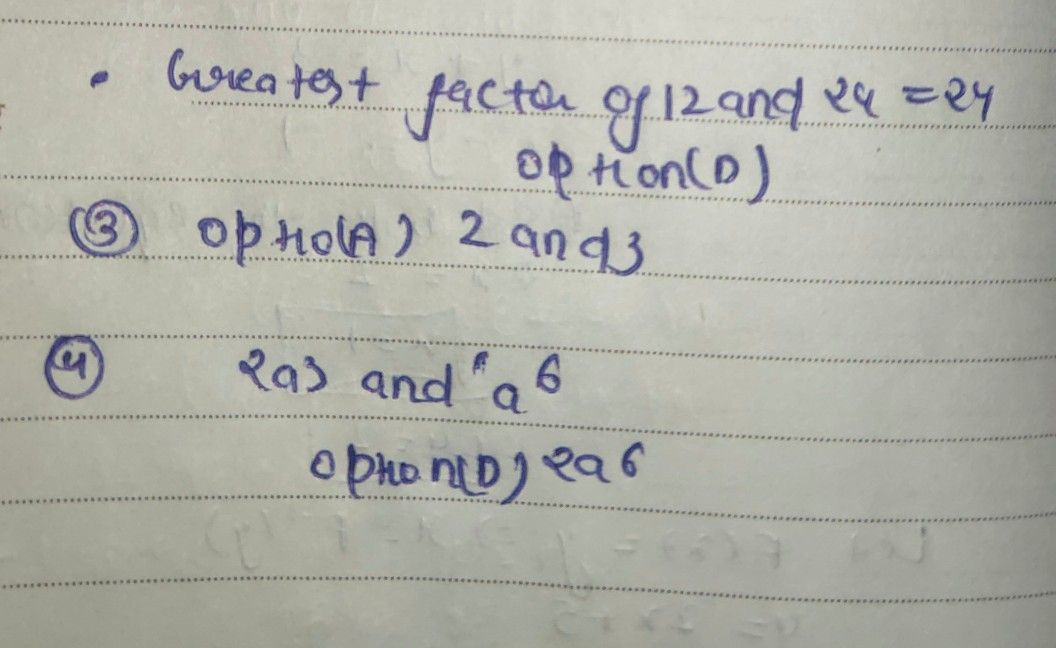Symbol
ProblemMATH 8 $Dircciim$ WRITTEN WORK NO. $1-θaa+|0x$ $koo5x$ the tetter of the correct answer White $k0a$ the chosen letter on the space provided bapre the number. $w4c$ is the $n0ccss$ of finding the factors of án expression is the reverse $Proccs$ of mC urltaitpiolincalaitzioanti? on D.Cntinsous A.factoring P. special product division $-2u$ What is the Breatest Common actor of 12and 24? $4.$ 2 $B$ 4 C. 12 D. 24 3 Which of the following pair of numbers has $a9<$ $/2$ $Q467$ A. 2 and 3 Be C. Gand 10 D. 12 4 What is the $C_{9c}$ $e3$ and a? A a B. $2a^{GCF°}c^{f}$ $3$ D. $a^{2}6^{a^{2}69,4nd}a^{2}60$ $6$ $-5$ SWhat is the GCF of $a^{5}b^{8}$ $a^{12}b^{2}$ $a^{4}b^{6}$ D.066 D.
7th-9th grade
Other
Search count: 106
SolutionQanda teacher - Askhina